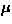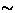Next: 2.2.4 Counter/muon spin geometries Up: 2.2 Experimental setup for Previous: Cryostats

### 2.2.3 Electronics and data handling

The signals from the muon/positron counters are shaped to a logical pulse using the CFD (Constant Fraction Discriminator), which is commonly used in nuclear experiments. The logical pulses are handled by the circuits shown in Fig.12, in order to take the time spectrum of thedecay. The main scheme of the electronics follows the thick solid lines; the muon signal' starts the stopwatch called TDC (Time to Digital Converter) and one of the positron signals' stops it. The elapsed time between these two events is stored in the histogram memory, along with the information of which positron counter gave the stop signal. The histogram memory is read by the data-taking VAX-computer typically every 5 minutes.The circuit is equipped with `pile-up rejection' logic (thin solid line in Fig.12), which is necessary forSR measurements using a continuous beam, as at TRIUMF. This circuit rejects situations where more than two muons reside in the apparatus within the experimental time window; in this situation, there is no way of knowing which muon decays to a given positron, and therefore, the data must be thrown away. The gate generator with the dashed lines determines the time range of interest for the measurements (12s). This time range is usually set500 ns shorter than the time window for the pileup rejection.Using the electronics described as above, as well as the muon-beam and the spectrometer, one obtains the time spectrum of thedecay, as shown in Fig.13. There is, however, random background overlapping the spectrum, an inevitable feature of continuous-beam experiments. Usually, one assumes that the background is time independent, and estimates its level from the spectrum at the t<0 range (the inset of Fig.13). The estimated background is evenly subtracted from the measured spectrum. The existence of the background sets the technical upper-limit of the experimental time range (12s) available for the continuous-beamSR method.

After subtracting the background, one calculates the corrected asymmetry (eq.12), and obtains the time evolution of the muon spin polarization, as shown in Fig.9b.Next: 2.2.4 Counter/muon spin geometries Up: 2.2 Experimental setup for Previous: Cryostats